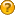###Author Topic: movement of the aircraft  (Read 870 times)

####EagleAlex

• byte
•• Posts: 1##### movement of the aircraft
« on: October 31, 2012, 08:29:34 am »
I try to create the engine of the aircraft (game) and all went well until moment when I had to move the plane a bit in the other direction. I need to rotate the nose of the plane and fly down there where he was looking ,but the plane went in the coordinates X ie forward as she possibly could if I had not turned. Tell me how can I make the plane fly out where to turn.

Rotation carries out
Code: [Select]
` if (move_left) { model.rotateY(-0.01f); } if (move_right) { model.rotateY(0.01f); }`Moving backward/forward
Code: [Select]
` if (move_backward) { model.translate(v.x, v.y, v.z+speed); } if (move_forvard) { model.translate(v.x, v.y, v.z-speed); } `
Code: [Select]
`private void move() { SimpleVector v = model.getZAxis(); SimpleVector s = model.getYAxis(); SimpleVector t = model.getXAxis(); if (forward) { camZ-=5; } if (backward) { camZ+=5; } if (move_left) { model.rotateY(-0.01f); } if (move_right) { model.rotateY(0.01f); } if (move_backward) { model.translate(v.x, v.y, v.z+speed); } if (move_forvard) { model.translate(v.x, v.y, v.z-speed); } if (fly_up) { model.translate(s.x, s.y-speed, s.z); } if (fly_down) { model.translate(s.x, s.y+speed, s.z); } if (to_vertical) { model.translate(t.x+speed , t.y , t.z); } if (to_horizontal) { model.translate(t.x-speed , t.y , t.z); } }`
« Last Edit: October 31, 2012, 08:32:27 am by EagleAlex »

####EgonOlsen

••